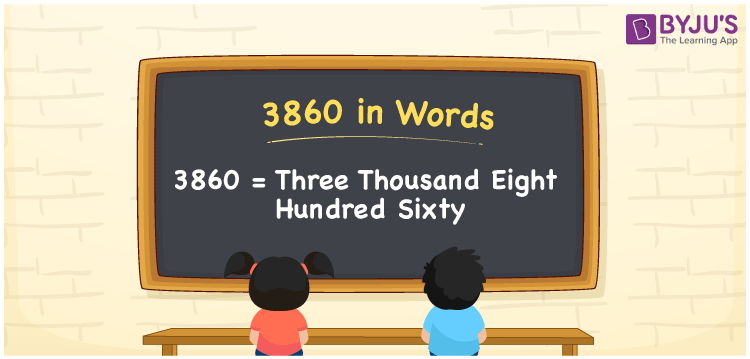# 3860 in Words

The number 3860 in words is “Three Thousand Eight Hundred Sixty”. The number 3860 is a cardinal number, which helps to count things. The place value system plays a major role in writing the number name. For example, if you spent 3860 in a month, you can write “I spent Rs. Three thousand eight hundred sixty”. This article will discuss the spelling of 3860 in English words and its procedure in detail.

 3860 in Words: Three Thousand Eight Hundred Sixty. Three Thousand Eight Hundred Sixty in Numerical Form: 3860.

## 3860 in English Words## How to Write 3860 in Words?

We will use the place value table to write the number name 3860. Go through the below table to learn the place values of 3860.

 Thousands Hundreds Tens Ones 3 8 6 0

The expanded form of 3860 is as follows:

= 3 × Thousand + 8 × Hundred + 6 × Ten + 0 × One

= 3 × 1000 + 8 × 100 + 6 × 10

= 3000 + 800 + 60

= 3860

= Three thousand eight hundred sixty

Hence, 3860 in words is three thousand eight hundred sixty.

3860 in words – Three thousand eight hundred sixty

Is 3860 an odd number? – No

Is 3860 an even number? – Yes

Is 3860 a perfect square number? – No

Is 3860 a perfect cube number? – No

Is 3860 a prime number? – No

Is 3860 a composite number? – Yes

## Frequently Asked Questions on 3860 in Words

Q1

### How to write 3860 in words?

3860 in words is three thousand eight hundred sixty.

Q2

### Simplify 3800 + 60, and express it in words.

Simplifying 3800 + 60, we get 3860. Hence, 3860 in words is three thousand eight hundred sixty.

Q3

### Convert three thousand eight hundred sixty into numbers.

Three thousand eight hundred sixty in numbers is 3860.